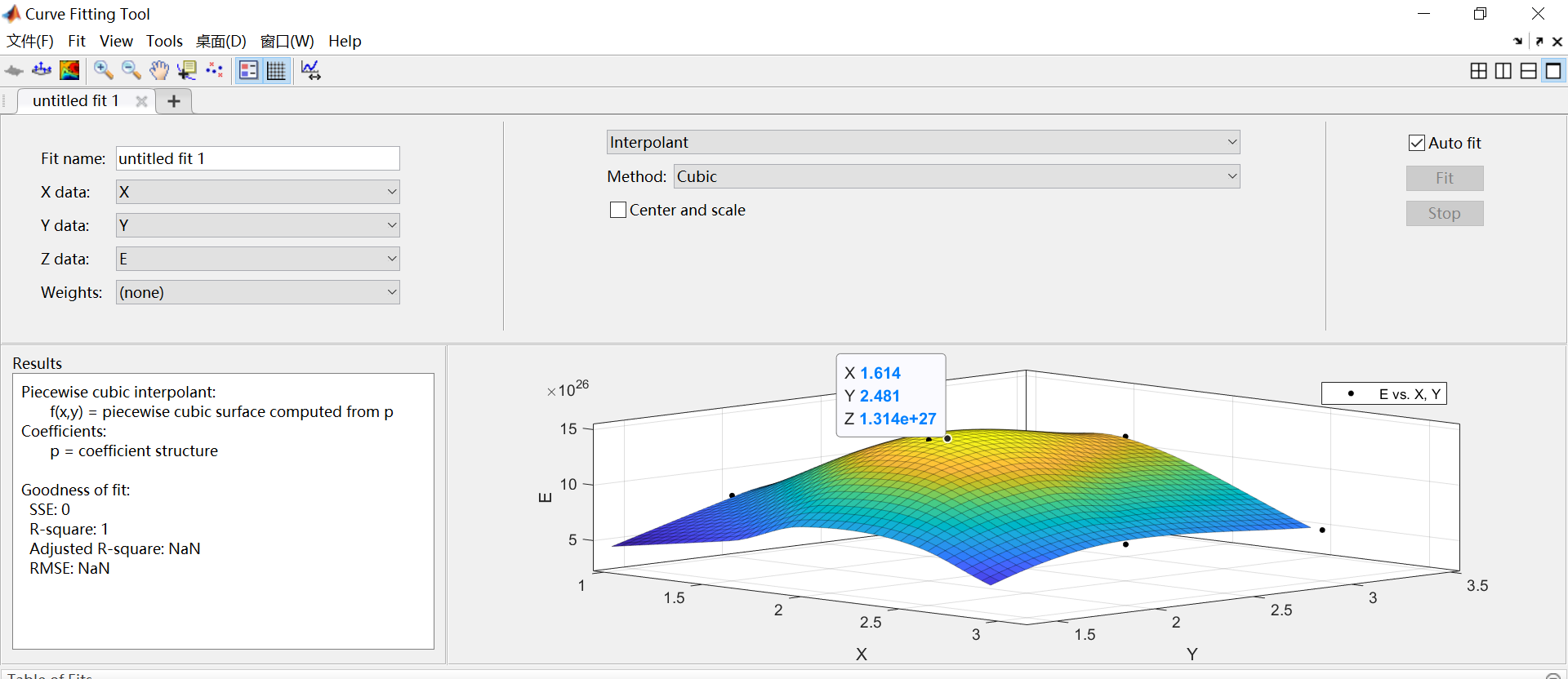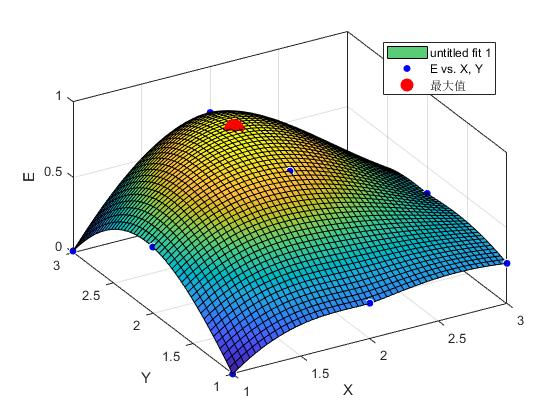qq_19519033 2021-10-27 11:35 采纳率: 72.7%

# 请问怎么查看cftool工具箱拟合出的曲面的数据呢？（curve fitting tool）• 写回答

#### 2条回答默认 最新

•joel_1993 2021-10-27 11:42
关注

工具箱生成的数值都可以从命令行直接获取：

``````E=rand(3);%E你自己取
[X,Y] = meshgrid(1:3);
[xData, yData, zData] = prepareSurfaceData( X, Y, E );
% Set up fittype and options.
ft = 'biharmonicinterp';
% Fit model to data.
[fitresult, gof] = fit( [xData, yData], zData, ft, 'Normalize', 'on' );
% Plot fit with data.
figure( 'Name', 'untitled fit 1' );
h = plot( fitresult, [xData, yData], zData );

% Label axes
xlabel X
ylabel Y
zlabel E
grid on
view( -20.0, 6.3 );
[X,Y] = meshgrid(1:0.01:3);
Z = fitresult(X,Y);
[~,I] = maxk(Z(:),1);
hold on
h(3)=plot3(X(I),Y(I),Z(I),'ro','markersize',14,'markerfacecolor','r');
legend( h, 'untitled fit 1', 'E vs. X, Y','最大值', 'Location', 'NorthEast' );
fprintf('X=%f,Y=%f,Zmax=%f\n',X(I),Y(I),Z(I))
``````最后类似于这种，有帮助望采纳呢

本回答被题主选为最佳回答 , 对您是否有帮助呢?
评论